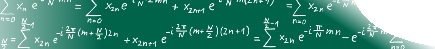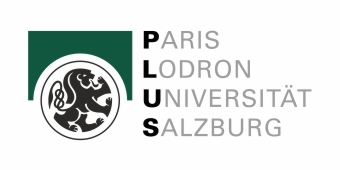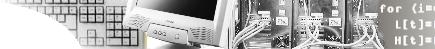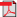home  |   research   |  members   |  projects  |  publications   |  conferences of interest   |  downloads   |  contact  |  intern

An Effective and Efficient Visual Quality Index Based on Local Edge Gradients

This is "The Multimedia Signal Processing and Security Lab", short WaveLab, website. We are a research group at the Computer Sciences Department of the University of Salzburg headed by Andreas Uhl. The short name "WaveLab" already indicates that wavelets are among our favorite tools - we have 15 years of experience in this area. Our research is focused on Multimedia Security including Watermarking, Image and Video Compression, Medical Image Classification, and Biometrics.

PaperHeinz Hofbauer, Andreas Uhl, "An Effective and Efficient Visual Quality Index based on Local Edge Gradientas", In IEEE 3rd European Workshop on Visual Information Processing, pp. 6pp., Paris, France, July 4-6,2011

Errata

In step 5 of the algorihtm the equation should read:
$ES\left(I,O\right)=\frac{4}{WH}\sum _{x=1}^{\frac{W}{2}}\sum _{y=1}^{\frac{H}{2}}\left(LE\left({I}_{0},{O}_{0},x,y\right)*\frac{1}{3}\sum _{i=1}^{3}LED\left({I}_{i},{O}_{i},x,y\right)\right).$

VQI v0.3